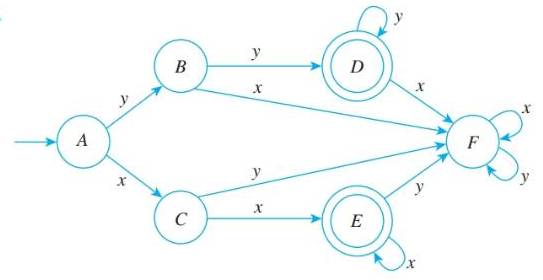Chapter 12.2, Problem 5ES### Discrete Mathematics With Applicat...

5th Edition
EPP + 1 other
ISBN: 9781337694193

#### Solutions

Chapter
Section### Discrete Mathematics With Applicat...

5th Edition
EPP + 1 other
ISBN: 9781337694193
Textbook Problem
1 views

# In 2—7, a finite-state automaton is given by a transition diagram. For each automaton: a. Find its states. b. Find its input symbols. C. Find its initial state. d. Find its accepting states. e. Write its annotated next-state table. 5To determine

(a)

For given automaton, find its states.

Explanation

Given information:

A finite-state automaton is given by a transition diagram.

To determine

(b)

For given automaton, find its input symbols.

To determine

(c)

For given automaton, find its initial state.

To determine

(d)

For given automaton, find its accepting states.

To determine

(e)

For given automaton, write its annotated next-state table.

### Still sussing out bartleby?

Check out a sample textbook solution.

See a sample solution

#### The Solution to Your Study Problems

Bartleby provides explanations to thousands of textbook problems written by our experts, many with advanced degrees!

Get Started